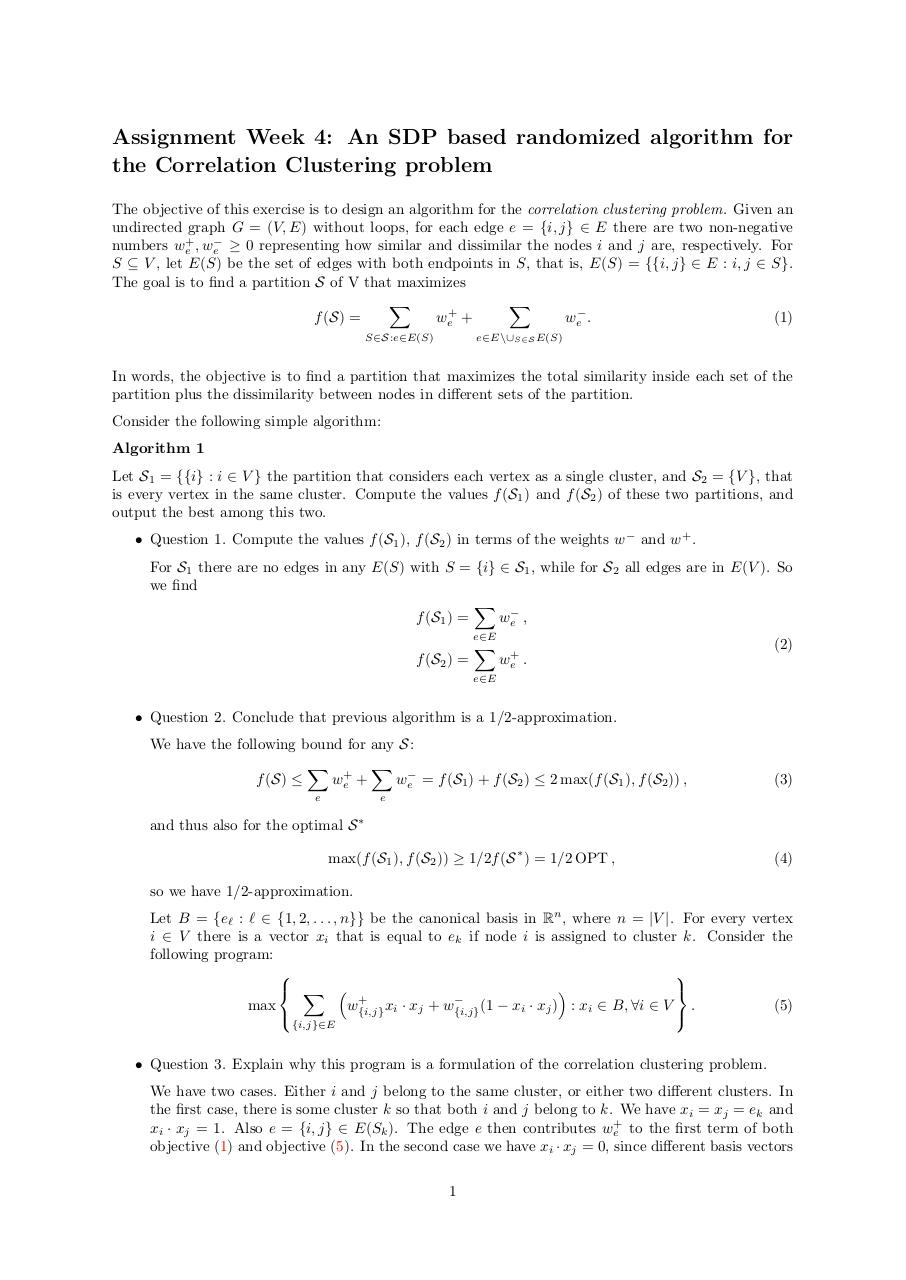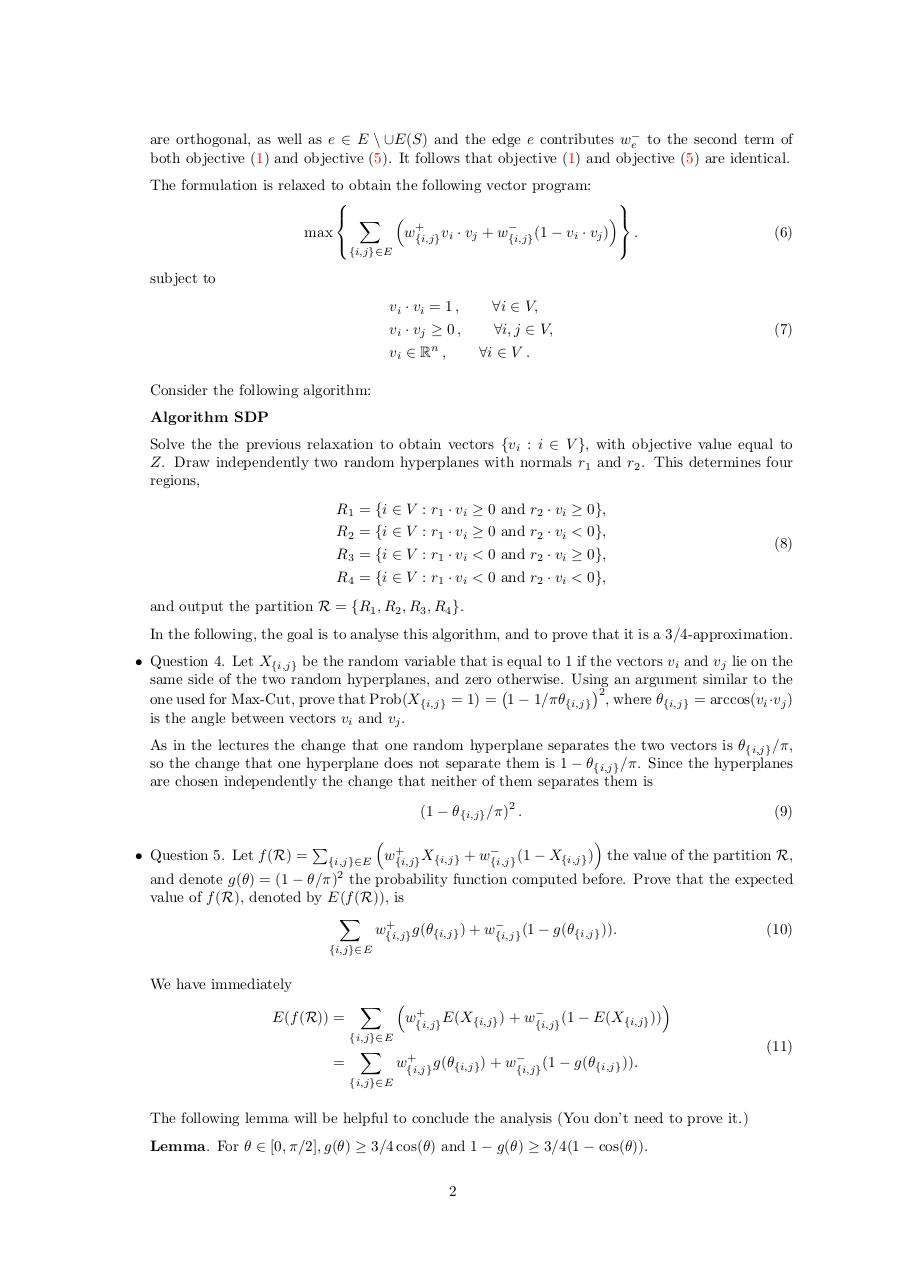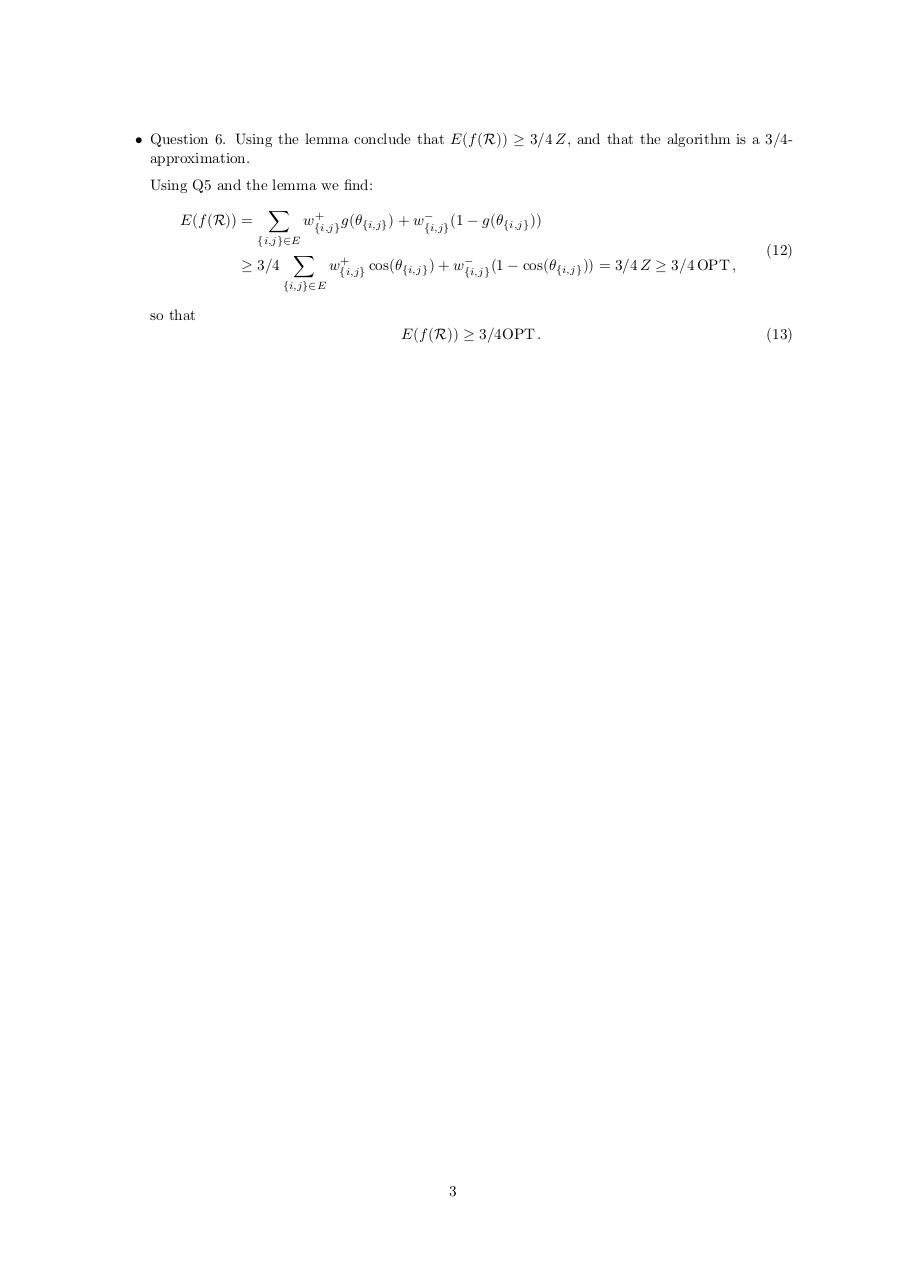# week4 .pdf

### File information

Original filename: week4.pdf

This PDF 1.5 document has been generated by LaTeX with hyperref package / pdfTeX-1.40.13, and has been sent on pdf-archive.com on 20/02/2016 at 19:01, from IP address 81.164.x.x. The current document download page has been viewed 415 times.
File size: 149 KB (3 pages).
Privacy: public file

week4.pdf (PDF, 149 KB)

### Document preview

Assignment Week 4: An SDP based randomized algorithm for
the Correlation Clustering problem
The objective of this exercise is to design an algorithm for the correlation clustering problem. Given an
undirected graph G = (V, E) without loops, for each edge e = {i, j} ∈ E there are two non-negative
numbers we+ , we− ≥ 0 representing how similar and dissimilar the nodes i and j are, respectively. For
S ⊆ V , let E(S) be the set of edges with both endpoints in S, that is, E(S) = {{i, j} ∈ E : i, j ∈ S}.
The goal is to find a partition S of V that maximizes
X
X
f (S) =
we+ +
we− .
(1)
S∈S:e∈E(S)

e∈E\∪S∈S E(S)

In words, the objective is to find a partition that maximizes the total similarity inside each set of the
partition plus the dissimilarity between nodes in different sets of the partition.
Consider the following simple algorithm:
Algorithm 1
Let S1 = {{i} : i ∈ V } the partition that considers each vertex as a single cluster, and S2 = {V }, that
is every vertex in the same cluster. Compute the values f (S1 ) and f (S2 ) of these two partitions, and
output the best among this two.
• Question 1. Compute the values f (S1 ), f (S2 ) in terms of the weights w− and w+ .
For S1 there are no edges in any E(S) with S = {i} ∈ S1 , while for S2 all edges are in E(V ). So
we find
X
f (S1 ) =
we− ,
e∈E

f (S2 ) =

X

we+ .

(2)

e∈E

• Question 2. Conclude that previous algorithm is a 1/2-approximation.
We have the following bound for any S:
X
X
f (S) ≤
we+ +
we− = f (S1 ) + f (S2 ) ≤ 2 max(f (S1 ), f (S2 )) ,
e

(3)

e

and thus also for the optimal S ∗
max(f (S1 ), f (S2 )) ≥ 1/2f (S ∗ ) = 1/2 OPT ,

(4)

so we have 1/2-approximation.
Let B = {e` : ` ∈ {1, 2, . . . , n}} be the canonical basis in Rn , where n = |V |. For every vertex
i ∈ V there is a vector xi that is equal to ek if node i is assigned to cluster k. Consider the
following program:

 X 


+

max
w{i,j}
xi · xj + w{i,j}
(1 − xi · xj ) : xi ∈ B, ∀i ∈ V .
(5)

{i,j}∈E

• Question 3. Explain why this program is a formulation of the correlation clustering problem.
We have two cases. Either i and j belong to the same cluster, or either two different clusters. In
the first case, there is some cluster k so that both i and j belong to k. We have xi = xj = ek and
xi · xj = 1. Also e = {i, j} ∈ E(Sk ). The edge e then contributes we+ to the first term of both
objective (1) and objective (5). In the second case we have xi · xj = 0, since different basis vectors
1

are orthogonal, as well as e ∈ E \ ∪E(S) and the edge e contributes we− to the second term of
both objective (1) and objective (5). It follows that objective (1) and objective (5) are identical.
The formulation is relaxed to obtain the following vector program:

 X 

+

max
w{i,j}
vi · vj + w{i,j}
(1 − vi · vj )
.

(6)

{i,j}∈E

subject to
vi · vi = 1 ,

∀i ∈ V,

vi · vj ≥ 0 ,

∀i, j ∈ V,

n

vi ∈ R ,

(7)

∀i ∈ V .

Consider the following algorithm:
Algorithm SDP
Solve the the previous relaxation to obtain vectors {vi : i ∈ V }, with objective value equal to
Z. Draw independently two random hyperplanes with normals r1 and r2 . This determines four
regions,
R1 = {i ∈ V : r1 · vi ≥ 0 and r2 · vi ≥ 0},
R2 = {i ∈ V : r1 · vi ≥ 0 and r2 · vi &lt; 0},

(8)

R3 = {i ∈ V : r1 · vi &lt; 0 and r2 · vi ≥ 0},
R4 = {i ∈ V : r1 · vi &lt; 0 and r2 · vi &lt; 0},
and output the partition R = {R1 , R2 , R3 , R4 }.

In the following, the goal is to analyse this algorithm, and to prove that it is a 3/4-approximation.
• Question 4. Let X{i,j} be the random variable that is equal to 1 if the vectors vi and vj lie on the
same side of the two random hyperplanes, and zero otherwise. Using an argument similar to the
2
one used for Max-Cut, prove that Prob(X{i,j} = 1) = 1 − 1/πθ{i,j} , where θ{i,j} = arccos(vi ·vj )
is the angle between vectors vi and vj .
As in the lectures the change that one random hyperplane separates the two vectors is θ{i,j} /π,
so the change that one hyperplane does not separate them is 1 − θ{i,j} /π. Since the hyperplanes
are chosen independently the change that neither of them separates them is
(1 − θ{i,j} /π)2 .
• Question 5. Let f (R) =



P

{i,j}∈E

(9)


+

w{i,j}
X{i,j} + w{i,j}
(1 − X{i,j} ) the value of the partition R,

and denote g(θ) = (1 − θ/π)2 the probability function computed before. Prove that the expected
value of f (R), denoted by E(f (R)), is
X
+

w{i,j}
g(θ{i,j} ) + w{i,j}
(1 − g(θ{i,j} )).
(10)
{i,j}∈E

We have immediately
E(f (R)) =

X 

+

w{i,j}
E(X{i,j} ) + w{i,j}
(1 − E(X{i,j} ))



{i,j}∈E

=

X

+

w{i,j}
g(θ{i,j} ) + w{i,j}
(1 − g(θ{i,j} )).

{i,j}∈E

The following lemma will be helpful to conclude the analysis (You don’t need to prove it.)
Lemma. For θ ∈ [0, π/2], g(θ) ≥ 3/4 cos(θ) and 1 − g(θ) ≥ 3/4(1 − cos(θ)).
2

(11)

• Question 6. Using the lemma conclude that E(f (R)) ≥ 3/4 Z, and that the algorithm is a 3/4approximation.
Using Q5 and the lemma we find:
X
+

E(f (R)) =
w{i,j}
g(θ{i,j} ) + w{i,j}
(1 − g(θ{i,j} ))
{i,j}∈E

≥ 3/4

X

+

w{i,j}
cos(θ{i,j} ) + w{i,j}
(1 − cos(θ{i,j} )) = 3/4 Z ≥ 3/4 OPT ,

(12)

{i,j}∈E

so that
E(f (R)) ≥ 3/4OPT .

3

(13)#### HTML Code

Copy the following HTML code to share your document on a Website or Blog

#### QR Code### Related keywords